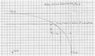# A Puzzle: Find an Ellipse Tangent to a Circle

• quantumdude

#### quantumdude

Staff Emeritus
Gold Member
I was asked to look at a problem (not homework) in which a tangent ellipse is to be found for a circle. This puzzle is turning out to be more than I bargained for. See the attached image because hey, a picture's worth a thousand words.

The givens in this problem are to be the radius ##R_i## of the circle, the eccentricity ##e## of the ellipse, and the distance ##R_o## from the center of the circle to either of the lines tangent to the ellipse. I also have that the tangent ellipse is to be centered at the origin and that it has a horizontal major axis.

I am to find the semiaxes ##a## and ##b## of the ellipse, the center ##(h,k)## of the circle, the point ##\left(x_0,y_0\right)## of tangency, and the slope ##m_t## of the circle/ellipse at the point of tangency. The horizontal and vertical tangents to the ellipse are ##y=b## and ##x=a##, respectively, but I don't know exactly where they are.

I have developed a little bit of shorthand to make the equations simpler. I've let ##d=\sqrt{1-e^2}## and ##m_n=-1/m_t##. The latter is the slope of the normal line that passes through ##\left(x_0,y_0\right)##. I also noted the line that passes through ##(h,k)## and ##(a,b)## has slope 1, so I thought it could be of interest. I call it "the 45 degree line". So the equations of the ellipse, circle, normal line, and 45 degree line are, respectively:

$$\frac{x^2}{a^2}+\frac{y^2}{d^2a^2}=1$$
$$(x-h)^2+(y-k)^2=R_i^2$$
$$y=m_n(x-h)+k$$
$$y=x-a+da$$

Here are the relationships that I have derived so far.

• Because the center of the circle is to be the same distance from either of the tangent lines ##y=b## or ##x=a##, I have ##h=a-R_o## and ##k=da-R_o##.
• Using the equation of the ellipse and its implicit derivative, I have ##x_0=\frac{a}{\sqrt{1+m_n^2d^2}}## and ##y_0=\frac{m_nd^2a}{\sqrt{1+m_n^2d^2}}##.
• Plugging the last two relations into ##m_n=\frac{y_0-k}{x_0-h}## I obtained a 4th degree polynomial equation for ##m_n##. Only one problem: it also contains the unknown quantity ##a##. I'll post the equation if anyone really wants to see it, but it's really not necessary. Solving that equation would be the final step, and I would throw it into Maple anyway.
I feel like I'm on the verge of solving this problem. If I could just find any of the unknowns ##a,b,h,## or ##k##, I could find all of the others and this thing would be done. But I've been chasing my own tail for so long that I just can't see my way out of it. I'm hoping that a fresh pair of eyes will help. Thanks!

#### Attachments

•Snip.JPG
35.1 KB · Views: 580
If mn = 1 are you in business?

Yes, and I considered that too. If ##m_n=1## then the normal line *is* the 45-degree line, which certainly makes the math easier. However, if that's the case then there isn't a solution for every choice of ##e,R_i##, and ##R_o##. In fact I don't know if there's a solution for any choice of those variables if ##m_n=1##.

Yet, mn = 1 drops out of what you've posted. Can't say I'm comfy with the implications, and wasn't up all night mulling the geometry, but it's got me going just the same.

there isn't a solution for every choice of e,R i e,R_i, and R o R_o.
I've been confining the problem statement to a "given" circle with a "given" center, which it is not. You get to move the circle, so there is a range of eccentricity that is possible.

##m_n=1## does not drop out of those equations. What does drop out is this:

##d^2\left(a-R_o\right)^2m_n^4-2d^2\left(a-R_o\right)\left(da-R_o\right)m_n^3+\left(\left(a-R_o\right)^2+d^2\left(da-R_o\right)^2-e^4a^2\right)m_n^2-2\left(a-R_o\right)\left(da-R_o\right)m_n##
##+\left(da-R_o\right)^2=0##

If I had ##a## (or ##h,k,## or ##b##, which would be just as good), I could evaluate the coefficients of this equation and chuck it into Maple to solve for ##m_n##. Eccentricity is one of the givens. That's what is making this so hard. If ##a## and ##b## were both given seperately, this would be an exercise in freshman calculus.

Lost the tangent point subscript translating between equation editors. Back to the drawing board.